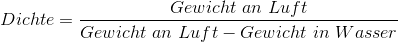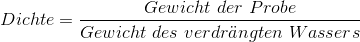## Archimedes' principle

Process discovered by Archimedes to determine the density of solid bodies.

This process is still used today to determine the density of metal, cast or melt specimens. This process is based on weighing a specimen the weigh of which is once determined in air and that is then weighted completely emerged in water. The lifting force corresponds to the fluid volume displaced by the body. As the density of water at room temperature is almost exactly 1 g/cm³, the measured lifting force (difference between the weight in air and the weight under water) corresponds to the volume V of the body in cm³ if the weights are determined in grams (Fig. 1). The density of the specimen in g/cm³ can be easily calculated from the relation ρ=m/V and respectively according to Eq. 1 .

Eq. 1:This weighing arrangement is also referred to as hydrostatic scale and is also applied in hydrometers (Fig. 2) with an electronic weigh-bridge. A beaker is filled with water and positioned on the weigh-bridge and the weigh is exactly set zero grams. The specimen is hanging on a thread and is submerged in the water while the scale only displays the weight of the water displaced by the specimen. This weight corresponds to the difference between the specimen weight in air and under water. Afterwards, the specimen is lowered to the ground of the beaker to determine its weight. If the weight is measured in grams, the density is defined according to Eq. 2:

Eq. 2: